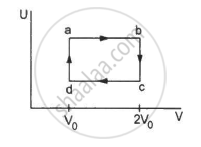Department of Pre-University Education, KarnatakaPUC Karnataka Science Class 11
Advertisement Remove all ads

# Figure Shows the Variation in the Internal Energy U with the Volume V of 2.0 Mol of an Ideal Gas in a Cyclic Process Abcda. the Temperatures of the Gas at B and C Are 500 K and 300 K Respectively. - Physics

Sum

Figure shows the variation in the internal energy U with the volume V of 2.0 mol of an ideal gas in a cyclic process abcda. The temperatures of the gas at b and c are 500 K and 300 K respectively. Calculate the heat absorbed by the gas during the process.Advertisement Remove all ads

#### Solution

Given: Number of moles of the gas, n = 2 moles

The system's volume is constant for lines bc and da.

Therefore,

∆V = 0

Thus, work done for paths da and bc is zero.

⇒ Wda = Wbc = 0

Since the process is cyclic, ∆U is equal to zero.

Using the first law, we get

∆W = ∆Q

∆W = ∆WAB + ∆WCD

Since the temperature is kept constant during lines ab and cd, these are isothermal expansions.

Work done during an isothermal process is given by

W = nRT

$\ln\frac{V_f}{V_i}$

If Vf and Vi are the initial and final volumes during the isothermal process, then

$W = n {RT}_1 ln\left( \frac{2 V_0}{V_0} \right) + n {RT}_2 ln\left( \frac{V_0}{2 V_0} \right)$

W = nR × 2.303 × log 2 × (500 − 300)

W = 2 × 8.314 × 2.303 × 0.301 × 200

W = 2305.31 J

Is there an error in this question or solution?
Advertisement Remove all ads

#### APPEARS IN

HC Verma Class 11, Class 12 Concepts of Physics Vol. 2
Chapter 4 Laws of Thermodynamics
Q 18 | Page 63
Advertisement Remove all ads
Advertisement Remove all ads
Share
Notifications

View all notifications

Forgot password?